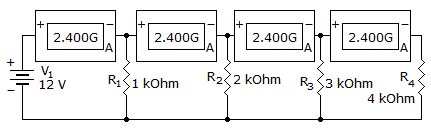# Electronics - Parallel Circuits - Discussion

Discussion Forum : Parallel Circuits - General Questions (Q.No. 10)
10.
Which component is shorted?R1
R2
R3
R4
Explanation:
No answer description is available. Let's discuss.
Discussion:
47 comments Page 1 of 5.

Kevin said:   8 years ago
The way I made sense of this problem is to think of the battery as a voltage source with and internal series resistor not shown.

Short circuit current is 2.4 amps Internal series resistance can be calculated, R=I/V, R=2.4 amps/12 Volts = 0.2 ohms.

The only way to get all 4 ammeters to read 2.4 amps is for R4 to be shorted.

Prakash said:   10 years ago
Hey guys G is not a Conductance if its conductance means they denote it as (mho) not by G we have to use its unit only after the values not variables its galvanometer I agree wit pankaj he is right G is galvanometer and it measure current. 2.4 A is in the next G so no current through the R1. Similarly, no current through R2, R3 and finally whole current through R4. So R4 is short circuited and others are open circuit.

Monali Trivedi said:   10 years ago
@P.Jayeshkumar.

I think each and every component has same junction point.

Imbesat alam said:   9 years ago
G is conductance and it is related to resistance as R=1/G.

Man singh said:   9 years ago
'A' in the box of 2.400 G confirms that it is Galvanometer.

Now since ammeter is connected in series & zero resistance is assumed offered by ammeter. So while solving we can ignore/ discard the galvanometer(ammeter) & redraw the circuit & then Solve it.

Total I = 25mA.

I1 Across R1 = 12mA.
I2 Across R2 = 6mA.
I3 Across R3 = 4mA.
I4 Across R4 = 1mA.

Recheck Total I = (I1+I2+I3+I4) = 25mA;

Short circuit means more current flows; while open circuit means no current flows or less current flows.

Max current is flowing through R1 & less through R4.

Basically current is flowing though all Resistances. Also Rating of Galvanometer is 2.4 that is much higher than 25mA (Total current) hence all Galvanometer are in working condition again confirming that current is flowing through all Resistances.

I would like to conclude that R1 is comparatively short circuit. But we all are trying to justify R4 because R4 is the option given in the answer series.

Milongo said:   9 years ago
When you look at this circuit its shown that the current has only one path (as it is indicated by the Galvanometer). Now understanding the meaning of open circuit which could be the same as a burnt resistor (no current flow). Then by FORCE. R4 is going to get all the current. Making the path of R4 a SHORT circuit.

Now, This does not means that R4, is not there, yes is there and after some time it is going to burn. Since it is to much current going through. This is a problem. Puff fire all over. Poor resistor R4.

Jessy said:   9 years ago
Just simply remember if R1>R2 in parallel circuit of resistance in this case current flow through R1 is less and R2 is more current.

Priya said:   8 years ago
@Cee.

Explains that current is highest in R4 but it is a obvious thing that current should be low in I4 because R4 = 12/R4.

Ravi said:   8 years ago
Short circuit means high circuit current. High circuit means low resistance.

As we all know, voltage is constant in parallel circuit. So I = V/R.

Current through R1 = 12mA.

Similarly through R2, R3 and R4 is 6mA, 4mA and 3mA.

Open circuit = No current or less current flows.

Short circuit = Large current flows.

Here R4 has Max current flow. So it would be short circuited.